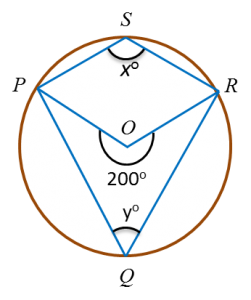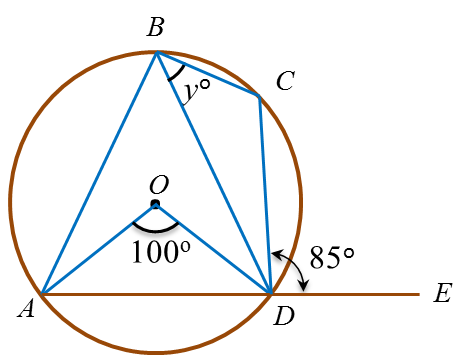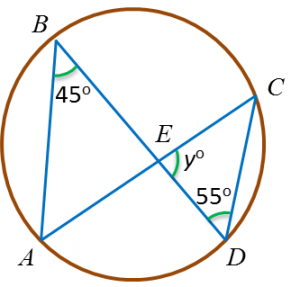# 3.2.1 Circles II, PT3 Practice

3.2.1 Circles II, PT3 Practice

Question 1:
(a) In Diagram below, KLMLPN and MPO are straight lines.

Find the value of x and of y.

(b) In the diagram below, O is the centre of the circle. Find the value of(i) x   (ii) y

Solution:
(a)
$\begin{array}{l}\angle LOM=\angle LNM={y}^{o}={44}^{o}\\ \therefore y=44\\ \angle NMO=\angle NLO={41}^{o}\\ {x}^{o}={180}^{o}-{80}^{o}-{41}^{o}\\ \text{}={59}^{o}\end{array}$

(b)(i)
2x = 200o
x = 100o

(b)(ii)
+ y = 180o
100o + y = 180o
y = 80o

Question 2:
(a) In Diagram below, AOC and BCD are straight lines with centre O.Find the value of y.

(b) In diagram below, ABCD is a circle with centre O and ADE is a straight line.Find the value of y.

Solution:
(a)
$\begin{array}{l}\angle AOB={45}^{o}×2={90}^{o}\\ {y}^{o}={180}^{o}-{90}^{o}={90}^{o}\end{array}$

(b)

$\begin{array}{l}\angle ABD=\frac{1}{2}×{100}^{o}={50}^{o}\\ \angle CDE=\angle ABC={85}^{o}\\ {y}^{o}+{50}^{o}={85}^{o}\\ \text{}{y}^{o}={35}^{o}\\ \text{}y=35\end{array}$

Question 3:
(a)  In diagram below, ABCD is a circle. AEC and BED are straight lines.Find the value of y.

(b) Diagram below shows a circle KLMN with centre O.Find the value of x.

Solution:
(a)
$\begin{array}{l}\angle ABE=\angle ACD={45}^{o}\\ {y}^{o}={180}^{o}-{45}^{o}-{55}^{o}\\ {y}^{o}={80}^{o}\\ y=80\end{array}$

(b)
$\begin{array}{l}\angle OML=\angle OLM={42}^{o}\\ \left({x}^{o}+{42}^{o}\right)+\angle KNM={180}^{o}\\ {x}^{o}+{42}^{o}+{110}^{o}={180}^{o}\\ {x}^{o}+{152}^{o}={180}^{o}\\ {x}^{o}={180}^{o}-{152}^{o}\\ {x}^{o}={28}^{o}\\ x=28\end{array}$

Question 5:
In diagram below, ABC and DEF are straight lines.Find the value of x and of y.

Solution: